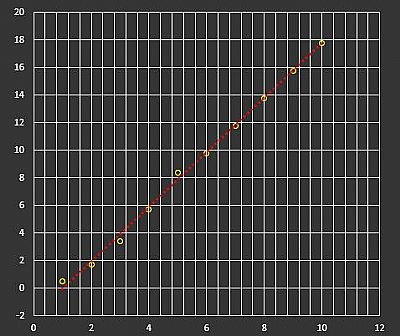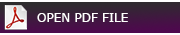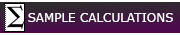## Least Squares Regression – How to fit a curve

Engineers and Scientists often need to carry out analysis of technical or experimental numerical data and find a relationship between the independent variables, often time periods or temperature change, and dependent variables such as fluid velocity or displacement being tested. If the relationship is linear such as a piece of machinery rotating at a constant acceleration then linear regression or interpolation can be used to determine the equation of a straight line.Graph of the equation of straight line: y = mx+c

Once the constants for the slope and intercept on the Y axis are known, the equation of the straight line can be used to find unknown values within the data range or extrapolate values outwith the data range.

When the relationship is precise such as machinery rotating at a constant acceleration then interpolation may provide the most accurate results. Regression is a more useful mathematical method for finding the relationship for dependent variables that are not precise, such as scattered variables. The line of best fit or trend line attempts to produce a fit that is closest to the points within the experimental data.

The data variables do not always fit within a straight line but can often be fitted to a curve. Polynomial, Lagrange or Spline interpolation can be used to obtain the best fit to the data. Alternatively, non-linear regression methods can be used. There are different types of non-linear regression curves, including logarithmic, exponential, power, polynomial and saturation growth curves. To find the best fit it may be necessary to try different methods to determine the closest fit for the data. The coefficient of determination R2 can sometimes be used to determine how close the regression predictions are to the real data points. When R2 is 1 the regression predictions are a perfect fit to the real data points.

Software such as IBM’s Statistical Package for Social Sciences* (SPSS) or Microsoft’s Excel* can be used for linear and non-linear regression analysis. Excel includes within its chart functions various trendline options, including exponential, linear, logarithmic, polynomial, power and moving average trendlines. Most of these trendline options allow the ability to display the equation and R2 value within the chart.

There are many different methods of finding the best fit to the data, but one method is least squares regression.  I have created eight SMath Studio* calculation sheets providing examples of how to obtain the best fit using the least squares methods. Please be aware I was an engineer not a mathematician and therefore I recommend anyone wanting further information on numerical data analysis should visit Wolfram MathWorld or other similar websites.

#### View PDF Files

Straight line interpolationLinear - Straight line regressionNon-linear exponential regressionNon-linear logrithmic regression3rd order polynominal cubic regressionNon-linear power regressionNon-linear saturation growth regression### Sources used and further reading

Least Squares Fitting - Wolfram MathWorld

Curve Fitting: Regression and Interpolation - D. C. McKinney - University of Texas at Austin

Numerical Methods: Least Squares Regression - C. Sert - Middle East Technical University

Linear Regression: Detailed View - Towards Data Science

Non-linear Regression - Wikipedia

Non-linear Least Squares Fitting - Wolfram MathWorld

* I have provided links on this page to commercial software packages for the benefit of visitors to my website. This is not an endorsement of any of these mathematical and statistical software packages.